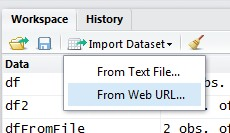# R - Join Data Frame (Merge)

## Syntax

``````merge(x = df1, y = df2, by = "Name")
merge(
x = df1,
y = df2,
by = intersect(names(x), names(y)),
by.x = by,
by.y = by,
all = FALSE, -- Shorthand for all.x and all.y
all.x = all,
all.y = all,
sort = TRUE,
suffixes = c(".x",".y"), -- To make unique the columns names
incomparables = NULL,
...
)```
```

where:

• the “by” parameters specifies the join column. Default: join by common variable names
• The by.x and by.y parameters must be used if the matching variables have different names
• the “all” parameters specifies which non matching row is returned.
• sort: Should the result be sorted on the by columns?

The default method coerces the arguments to data frames and calls the “data.frame” method.

## Join

### Inner

````merge(df1, df2) `
```

### Outer

#### Left outer

````merge(x = df1, y = df2, by = "Name", all.x = TRUE)`
```

#### Right outer

````merge(x = df1, y = df2, by = "Name", all.y = TRUE)`
```

#### Full OUter

``````merge(x = df1, y = df2, by = "Name", all.y = TRUE, all.x = TRUE)
# or
merge(x = df1, y = df2, by = "Name", all = TRUE)```
```

### Cross

````merge(x = df1, y = df2, by = NULL)`
```

## Documentation / Reference

Discover MoreR - Data frame Object

A data frame is a logical implementation of a table in a relational database A data frame inherits all the property and function of an object. It has a list of variables of the same number of rows with...